# Problem: On Time for the Exam

A student has to attend an exam at a particular time (for example at 9:30 am). They arrive in the exam room at a particular time of arrival (for example 9:40 am). It is considered that the student has arrived on time, if they have arrived at the time when the exam starts or up to half an hour earlier. If the student has arrived more than 30 minutes earlier, the student has come too early. If they have arrived after the time when the exam starts, they are late.

Write a program that inputs the exam starting time and the time of student's arrival, and prints if the student has arrived on time, if they have arrived early or if they are late, as well as how many hours or minutes the student is early or late.

## Input Data

Read the following four integers (one on each line) from the console:

• The first line contains exam starting time (hours) – an integer from 0 to 23.
• The second line contains exam starting time (minutes) – an integer from 0 to 59.
• The third line contains hour of arrival – an integer from 0 to 23.
• The fourth line contains minutes of arrival – an integer from 0 to 59.

## Output Data

Print the following on the first line on the console:

• "Late", if the student arrives later compared to the exam starting time.
• "On time", if the student arrives exactly at the exam starting time or up to 30 minutes earlier.
• "Early", if the student arrives more than 30 minutes before the exam starting time.

If the student arrives with more than one minute difference compared to the exam starting time, print on the next line:

• "mm minutes before the start" for arriving less than an hour earlier.
• "hh:mm hours before the start" for arriving 1 hour or earlier. Always print minutes using 2 digits, for example "1:05".
• "mm minutes after the start" for arriving more than an hour late.
• "hh:mm hours after the start" for arriving late with 1 hour or more. Always print minutes using 2 digits, for example "1:03".

## Sample Input and Output

Input Output Input Output
9
30
9
50
Late
20 minutes after the start
16
00
15
00
Early
1:00 hours before the start
9
00
8
30
On time
30 minutes before the start
9
00
10
30
Late
1:30 hours after the start
14
00
13
55
On time
5 minutes before the start
11
30
8
12
Early
3:18 hours before the start
Input Output
10
00
10
00
On time
11
30
10
55
Early
35 minutes before the start
11
30
12
29
Late
59 minutes after the start

## Hints and Guidelines

Let's solve the problem step by step.

### Processing the Input Data

According to the assignment, we expect four lines containing different integers to be passed. Examining the provided parameters, we can use the int type, as it is suitable for the expected values. We simultaneously read the input data and parse the string value to the selected data type for integer.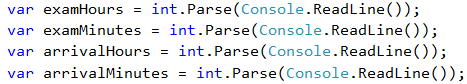Examining the expected output, we can create variables that contain the different output data types, in order to avoid using the so called "magic strings" in the code.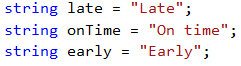### Calculating Exam Start Time and Student Arrival Time

After reading the input data, we can now start writing the logic for calculating the result. Let's first calculate the start time of the exam in minutes for easier and more accurate comparison.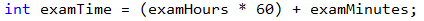Let's also calculate the student arrival time using the same logic.What remains is to calculate the difference between the two times, in order to determine when and what time compared to the exam time the student arrived at.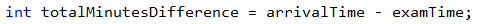### Checking If the Student Arrived on Time or They Were Late

Our next step is to do the required checks and calculations, and finally we will print the output. Let's separate the code into two parts:

• First, let's show when the student arrived – were they early, late or on time. In order to do that, we will use an if-else statement.
• After that, we will show the time difference, if the student arrives in a different time compared to the exam starting time.

In order to spare one additional check (else), we can, by default, assume that the student was late.

After that, according to the condition, we will check whether the difference in times is more than 30 minutes. If this is true, we assume that the student is early. If we do not match the first condition, we need to check if the difference is less than or equal to zero (<= 0), by which we are checking the condition whether the student arrived within the range of 0 to 30 minutes before the exam.

In all other cases we assume that the student was late, which we set as default, and no additional check is needed.### Calculating Time Difference

Finally, we need to understand and print what is the time difference between exam start time and student arrival time, as well as whether this time difference indicates time of arrival before or after the exam start.

We check whether the time difference is more than one hour, in order to print hours and minutes in the required format, or less than one hour, in order to print only minutes as a format and description.

We also need to do one more check – whether the time of student's arrival is before or after the exam start time.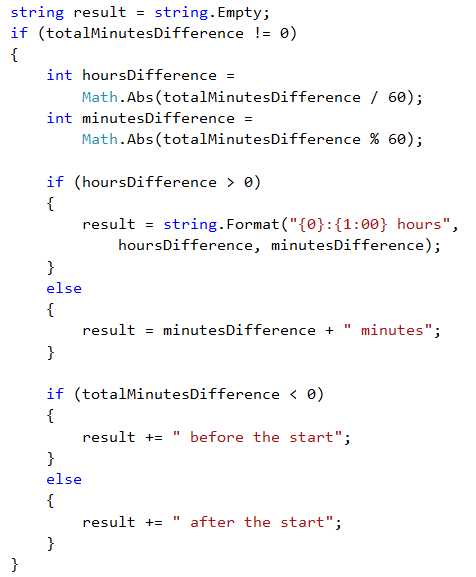### Printing the Result

Finally, what remains is to print the result on the console. According to the requirements, if the student arrived right on time (not even a minute difference), we do not need to print a second result. This is why we apply the following condition:Actually, for the purposes of the task, printing the result on the console can be done on a much earlier stage – during the calculations. This, however, is not a very good practice. Why?

Let's examine the idea that our code is not 10 lines, but 100 or 1000! One day, printing the result will not be done on the console, but will be written in a file or displayed as a web application. Then, how many places in the code you will make changes at, due to such a correction? Are you sure you won't miss some places?Always consider the code that contains logical calculations as a separate part, different from the part that processes the input and output data. It has to be able to work regardless of how the data is passed to it and where the result will be displayed.

## Testing in the Judge System

Test your solution here: https://judge.softuni.org/Contests/Practice/Index/509#0.